图解机器学习：神经网络和 TensorFlow 的文本分类已翻译 100%

oschina 投递于 2017/04/12 14:14 (共 13 段, 翻译完成于 04-19)

参与翻译 (3人) : 我是菜鸟我骄傲, 探花郎啊, 总长

1. TensorFlow 的工作原理

2. 什么是机器学习模型

3. 什么是神经网络

4. 神经网络如何学习

5. 如何操作数据并将其传递给神经网络

6. 如何运行模型并获得预测结果

TensorFlow

tf.Graph

• 一类 tf.Operation，表示计算单元

• 一类 tf.Tensor，表示数据单元（计算x+y的图）

import tensorflow as tf
x = tf.constant([1,3,6])
y = tf.constant([1,1,1])

import tensorflow as tf
x = tf.constant([1,3,6])
y = tf.constant([1,1,1])

import tensorflow as tf
my_graph = tf.Graph()
with my_graph.as_default():
x = tf.constant([1,3,6])
y = tf.constant([1,1,1])

tf.Session

tf.Session 对象封装了 Operation 对象的执行环境。Tensor 对象是被计算过的（从文档中）。为了做到这些，我们需要在 Session 中定义哪个图将被使用到：

import tensorflow as tf
my_graph = tf.Graph()
with tf.Session(graph=my_graph) as sess:
x = tf.constant([1,3,6])
y = tf.constant([1,1,1])

import tensorflow as tf
my_graph = tf.Graph()
with tf.Session(graph=my_graph) as sess:
x = tf.constant([1,3,6])
y = tf.constant([1,1,1])
result = sess.run(fetches=op)
print(result)
>>> [2 4 7]

预测模型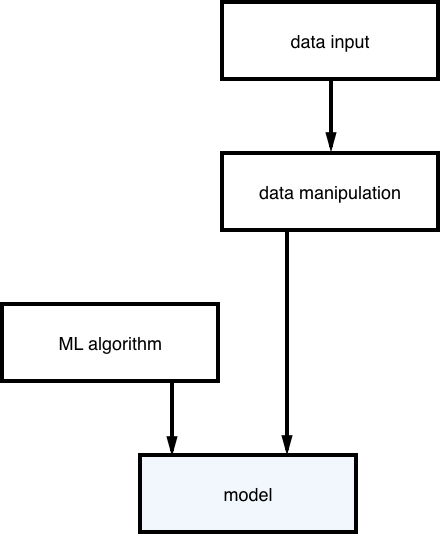（构建预测模型的过程）（预测工作流）

input: text, result: category

“我们知道正确的答案。该算法迭代的预测训练数据，并由老师纠正
” — Jason Brownlee

神经网络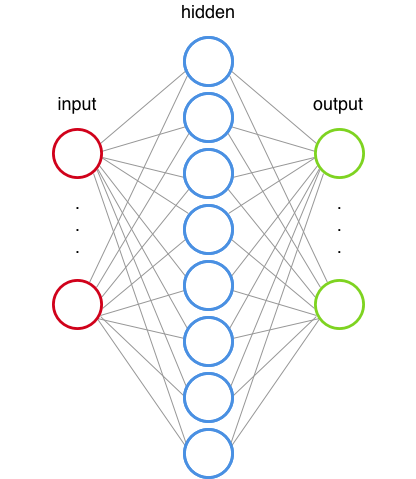（一个神经网络）

神经网络架构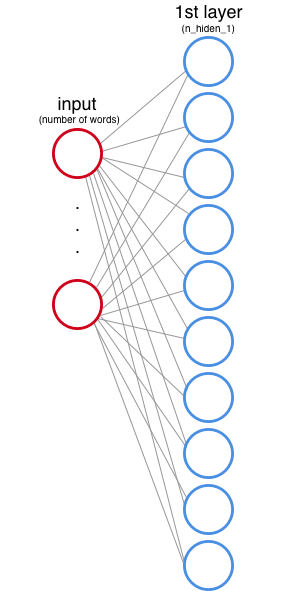（输入层和第一个隐藏层）

f(x) = max(0,x)  [输出 x 或者 0（零）中最大的数]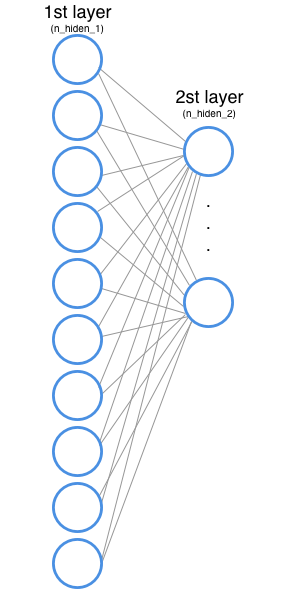(第一和第二隐藏层)

+-------------------+-----------+
|    category       |   value   |
+-------------------|-----------+
|      sports       |    001    |
|      space        |    010    |
| computer graphics |    100    |
|-------------------|-----------|

| 1.2                    0.46|
| 0.9   -> [softmax] ->  0.34|
| 0.4                    0.20|

# Network Parameters
n_hidden_1 = 10        # 1st layer number of features
n_hidden_2 = 5         # 2nd layer number of features
n_input = total_words  # Words in vocab
n_classes = 3          # Categories: graphics, space and baseball
def multilayer_perceptron(input_tensor, weights, biases):
layer_1_multiplication = tf.matmul(input_tensor, weights['h1'])
# Hidden layer with RELU activation
layer_2_multiplication = tf.matmul(layer_1_activation, weights['h2'])
# Output layer with linear activation
out_layer_multiplication = tf.matmul(layer_2_activation, weights['out'])

(我们将会在后面讨论输出层的激活函数)

神经网络怎么学习

tf.Variable

weights = {
'h1': tf.Variable(tf.random_normal([n_input, n_hidden_1])),
'h2': tf.Variable(tf.random_normal([n_hidden_1, n_hidden_2])),
'out': tf.Variable(tf.random_normal([n_hidden_2, n_classes]))
}
biases = {
'b1': tf.Variable(tf.random_normal([n_hidden_1])),
'b2': tf.Variable(tf.random_normal([n_hidden_2])),
'out': tf.Variable(tf.random_normal([n_classes]))
}

input values: x
weights: w
bias: b
output values: z
expected values: expected

James D. McCaffrey 写了一个精彩的解释，说明为什么这是这种类型任务的最佳方法。

# Construct model
prediction = multilayer_perceptron(input_tensor, weights, biases)
# Define loss
entropy_loss = tf.nn.softmax_cross_entropy_with_logits(logits=prediction, labels=output_tensor)
loss = tf.reduce_mean(entropy_loss)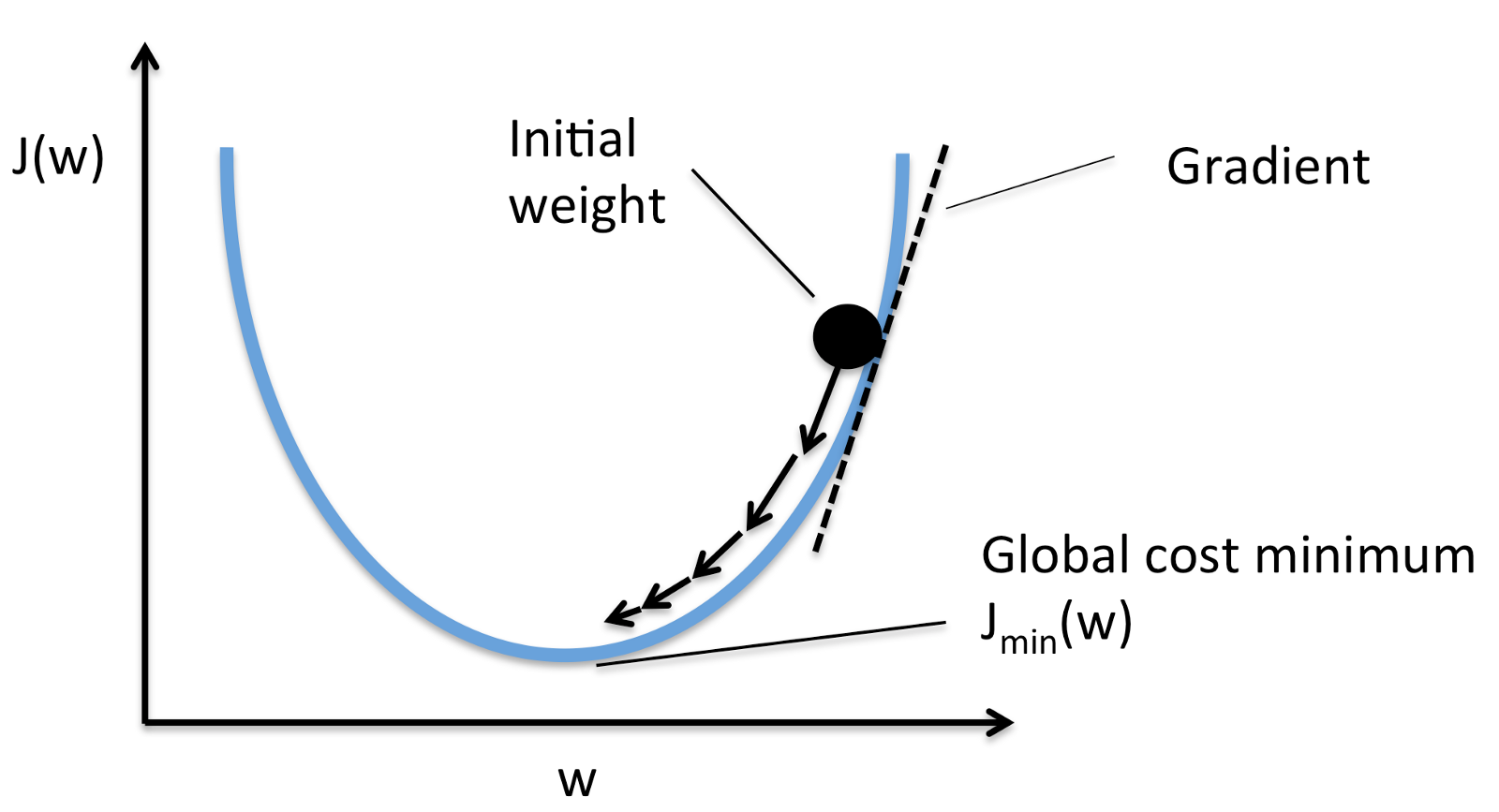learning_rate = 0.001
# Construct model
prediction = multilayer_perceptron(input_tensor, weights, biases)
# Define loss
entropy_loss = tf.nn.softmax_cross_entropy_with_logits(logits=prediction, labels=output_tensor)
loss = tf.reduce_mean(entropy_loss)

数据操作

1. 为每一个工作创建索引

2. 为每一个文本创建矩阵，在矩阵里，如果单词在文本中则值为 1，否则值为 0

import numpy as np    #numpy is a package for scientific computing
from collections import Counter
vocab = Counter()
text = "Hi from Brazil"#Get all wordsfor word in text.split(' '):
vocab[word]+=1
#Convert words to indexes
def get_word_2_index(vocab):
word2index = {}    for i,word in enumerate(vocab):
word2index[word] = i
return word2index
#Now we have an index
word2index = get_word_2_index(vocab)
total_words = len(vocab)
#This is how we create a numpy array (our matrix)
matrix = np.zeros((total_words),dtype=float)
#Now we fill the valuesfor word in text.split():
matrix[word2index[word]] += 1print(matrix)
>>> [ 1.  1.  1.]

matrix = np.zeros((total_words),dtype=float)
text = "Hi"for word in text.split():
matrix[word2index[word.lower()]] += 1print(matrix)
>>> [ 1.  0.  0.]

y = np.zeros((3),dtype=float)if category == 0:
y = 1.        # [ 1.  0.  0.]
elif category == 1:
y = 1.        # [ 0.  1.  0.]else:
y = 1.       # [ 0.  0.  1.]

评论(11)

get_batch()怎么做的？？？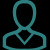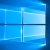引用来自“久永”的评论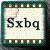引用来自“为为02”的评论